# Isosceles triangle

Calculate the perimeter of isosceles triangle with arm length 26 cm and base length of 21 cm.

Result

p =  73 cm

#### Solution:

$p = 2 \cdot 26 + 21 = 73 \ \text{ cm }$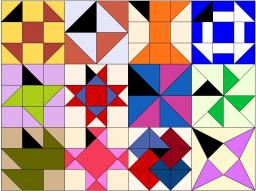Our examples were largely sent or created by pupils and students themselves. Therefore, we would be pleased if you could send us any errors you found, spelling mistakes, or rephasing the example. Thank you!

Leave us a comment of this math problem and its solution (i.e. if it is still somewhat unclear...):Be the first to comment!Tips to related online calculators
Do you want to convert length units?

## Next similar math problems:

1. Arm-legCalculate the length of the base of an isosceles triangle with a circumference 224 cm if the arm length is 68 cm.
2. Triangle ABCIn a triangle ABC side b measure 10 cm less than the side a and side b is half of the side c. Calculate the length of sides if the circumference of the triangle is 42 cm.
3. MedianThe median of the triangle LMN is away from vertex N 84 cm. Calculate the length of the median, which start at N.
4. AnglesThe triangle is one outer angle 158°54' and one internal angle 148°. Calculate the other internal angles of a triangle.
5. An equilateral triangle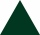The perimeter of an equilateral triangle is 33cm. How long is each side?
6. Triangle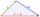Determine if it is possible to construct a triangle with sides 28 31 34 by calculation.
7. TriangleProve whether you can construct a triangle ABC, if a=9 cm, b=6 cm, c=10 cm.
8. 3-bracket 2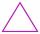May be the smallest angle in the triangle greater than 40°?
9. Triangle P2Can triangle have two right angles?
10. RectangleThe rectangle area is 182 dm2, its base is 14 dm. How long is the other side? Calculate its perimeter.
11. Athlete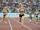How long length run athlete when the track is circular shape of radius 120 meters and an athlete runs five times in the circuit?
12. RhombusFind the length of each side of rhombus if the perimeter is 49 dm long.
13. Clock handsThe second hand has a length of 1.5 cm. How long does the endpoint of this hand travel in one day?
14. Mr. TolentinoMr. Tolentino own a piece of land, it measures 86.12 meters long 68.293 meters width. How much longer is the length than the width?
15. Relay-race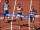Kate ran 170 meters at 29.8 seconds, Suzan at 29.3 seconds, Roza at 34 seconds and Žofka at 30.4 seconds. How long took to run 4x170 m relay-race?
16. Apartment areaAnton wanted to measure the area of the apartment. But he bought a meter only 1.5m long. Later he recalled that he had two meters long at 4.5m and 18m at home. How many times are home gauges longer than the meter he bought?
17. Trees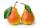In the orchard, 38 apple trees and 20 pear trees grew. How many trees there grow?